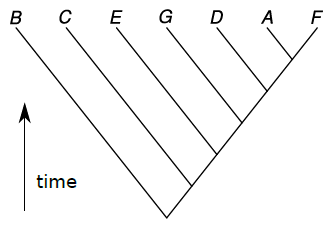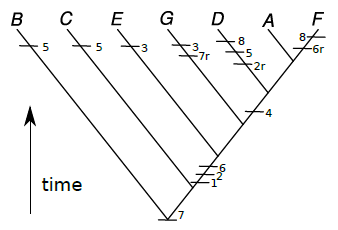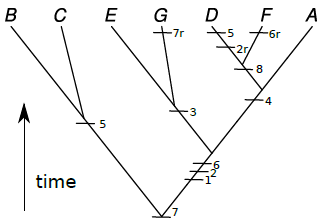# 10.4: Lets Practice! How to make a phylogeny tree

$$\newcommand{\vecs}{\overset { \rightharpoonup} {\mathbf{#1}} }$$ $$\newcommand{\vecd}{\overset{-\!-\!\rightharpoonup}{\vphantom{a}\smash {#1}}}$$$$\newcommand{\id}{\mathrm{id}}$$ $$\newcommand{\Span}{\mathrm{span}}$$ $$\newcommand{\kernel}{\mathrm{null}\,}$$ $$\newcommand{\range}{\mathrm{range}\,}$$ $$\newcommand{\RealPart}{\mathrm{Re}}$$ $$\newcommand{\ImaginaryPart}{\mathrm{Im}}$$ $$\newcommand{\Argument}{\mathrm{Arg}}$$ $$\newcommand{\norm}{\| #1 \|}$$ $$\newcommand{\inner}{\langle #1, #2 \rangle}$$ $$\newcommand{\Span}{\mathrm{span}}$$ $$\newcommand{\id}{\mathrm{id}}$$ $$\newcommand{\Span}{\mathrm{span}}$$ $$\newcommand{\kernel}{\mathrm{null}\,}$$ $$\newcommand{\range}{\mathrm{range}\,}$$ $$\newcommand{\RealPart}{\mathrm{Re}}$$ $$\newcommand{\ImaginaryPart}{\mathrm{Im}}$$ $$\newcommand{\Argument}{\mathrm{Arg}}$$ $$\newcommand{\norm}{\| #1 \|}$$ $$\newcommand{\inner}{\langle #1, #2 \rangle}$$ $$\newcommand{\Span}{\mathrm{span}}$$$$\newcommand{\AA}{\unicode[.8,0]{x212B}}$$

The instructor will explain phylogeny method on any appropriate example (Sesame Street creatures, random stuff from a classroom) but the following instruction will explain it on the example of imaginary planet:

Planet Aqua is entirely covered with shallow water. The ocean is inhabited with various flat organisms (see the figure). These creatures (let us call them “kubricks”) can photosynthesize and/or eat other organisms or their parts (which match with their mouths), and move (only if they have no stalks). We need to make a phylogeny of kubrick species A–G because kubrick H is an outgroup and by definifion, has only plesiomorphic (ancestral) characters.

1. Start with the group you need to classify, assign the name for every object (member of the group).

(This is already done, see above.)

2. Find as many characters as possible. Working number of characters is $$N\ge n+1$$ where $$n$$ is the number of objects. Therefore, in this example we need $$N\ge8$$. Review all characters and make the character list.

Note that plesiomorphies and autapomorphies (unique derived characters) do not help to make a tree so concentrate on finding synapomorphies, derived characters which unite two or more (but not all) objects.

For every character, guess its plesiomorphic (ancestral) and apomorphic (derived) states, either (1) with the help of outgroup, or (2) using the other methods like comparative anatomy, paleontology or embryology. Encode plesiomorphic character state as “0”, and apomorphic as “1”:Figure $$\PageIndex{1}$$: Kubricks.

Character list:

1. Mouth(s) presents 1, no mouth 0

2. One mouth 1, not 0

3. Triangle mouth 1, not 0

4. Square mouth 1, not 0

5. Stalk 1, no stalk 0

6. No photosynthesis 1, photosynthesis 0

7. Body big 1, body small 0

8. Mouth on top 1, no mouth on top 0

Note character #6: since outgroup is photosynthetic, we must make the absence of photosynthesis apomorphic state.

Make the Character Table where rows are names of objects, columns are characters, and each cell contains 0 or 1:

 1 2 3 4 5 6 7 8 Kubrick A 1 1 0 1 0 1 1 0 Kubrick B 0 0 0 0 1 0 1 0 Kubrick C 0 0 0 0 1 0 1 0 Kubrick D 1 0 0 1 1 1 1 1 Kubrick E 1 1 1 0 0 1 1 0 Kubrick F 1 1 0 1 0 0 1 1 Kubrick G 1 1 1 0 0 1 0 0

Without such table, we cannot calculate the length of our future tree (see below).

Note that outgroup is not here; this is because we already polarized our characters and therefore do not need outgroup anymore.

3. Start to draw the tree, preferably from the most ancestral (“primitive”) member (which has most zeroes), this allows to pinpoint the root of our tree.

Most ancestral member is the first branch. Continue to grow the tree attaching branches, preferably (but not necessarily) placing most similar objects closer. Actually, you may even attach members at random because this tree will be optimized anyway. All terminal branches should end with objects.Figure $$\PageIndex{2}$$
4. Look at the complete tree and label all apomorphies from the character table as tickmarks with labels. Now calculate the length of tree which is a number of evolutionary events, or simply number of tickmarks.Figure $$\PageIndex{3}$$ Length of tree is simply the number of tickmarks, so $$L=29$$ (this is exactly how many "ones" are in the table). This is a bit too much since good tree should have length $$L< 2N$$ where $$N$$ is the number of characters. In our case, $$L< 16$$ is strongly recommended.
5. You will now likely see ways to improve your tree! Start to think how to make the tree shorter. First, decrease length without altering tree. You can shift characters down, deeper in time. Then all descendants from this point should have this character. If some do not have it, you may introduce reversal, the loss of character. Label reversals with "r" letter, like "2r":Figure $$\PageIndex{4}$$ Length of this tree is the number of all tickmarks so $$L= 15$$ now.
6. Then start to alter the actual tree. The basic method is to prune (take off) any branch (except root) and attach it to another place, re-label tree and calculate the length again. If the length is shorter, this is more parsimonious tree. Try to find the most parsimonious (shortest) tree, i.e. the tree with as low number of tickmarks as possible. Do not forget that tree branches may rotate freely.Figure $$\PageIndex{5}$$ Now $$L= 12$$! This is likely the most parsimonious tree. If you find the shorter one, you will receive extra points!

This page titled 10.4: Lets Practice! How to make a phylogeny tree is shared under a Public Domain license and was authored, remixed, and/or curated by Alexey Shipunov.